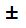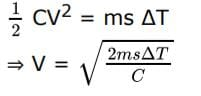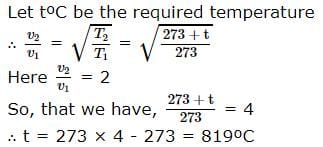JEE  >  BITSAT Physics Test - 2

# BITSAT Physics Test - 2

Test Description

## 40 Questions MCQ Test BITSAT Mock Tests Series & Past Year Papers | BITSAT Physics Test - 2

BITSAT Physics Test - 2 for JEE 2023 is part of BITSAT Mock Tests Series & Past Year Papers preparation. The BITSAT Physics Test - 2 questions and answers have been prepared according to the JEE exam syllabus.The BITSAT Physics Test - 2 MCQs are made for JEE 2023 Exam. Find important definitions, questions, notes, meanings, examples, exercises, MCQs and online tests for BITSAT Physics Test - 2 below.
Solutions of BITSAT Physics Test - 2 questions in English are available as part of our BITSAT Mock Tests Series & Past Year Papers for JEE & BITSAT Physics Test - 2 solutions in Hindi for BITSAT Mock Tests Series & Past Year Papers course. Download more important topics, notes, lectures and mock test series for JEE Exam by signing up for free. Attempt BITSAT Physics Test - 2 | 40 questions in 50 minutes | Mock test for JEE preparation | Free important questions MCQ to study BITSAT Mock Tests Series & Past Year Papers for JEE Exam | Download free PDF with solutions
 1 Crore+ students have signed up on EduRev. Have you?
BITSAT Physics Test - 2 - Question 1

### A transformer has turn ratio 100/1. If secondary coil has 4 amp current then current in primary coil is

Detailed Solution for BITSAT Physics Test - 2 - Question 1 We have the relation that
NsIs = NpIp
here, Ns = 1
Np= 100
Is = 4A
so, 1×4 = 100 × IP
Ip = 0.04
BITSAT Physics Test - 2 - Question 2

### In helium nucleus, there are

BITSAT Physics Test - 2 - Question 3

### Every series of hydrogen spectrum has an upper and lower limit in wavelength. The spectral series which has an upper limit of wavelength equal to 18752 Å is

BITSAT Physics Test - 2 - Question 4

If potential V=1000.5 Volt and current I = 100.2 amp are given to us. Then what will be the value of resistance

BITSAT Physics Test - 2 - Question 5

If an ammeter is connected in parallel to a circuit, it is likely to be damaged due to excess

Detailed Solution for BITSAT Physics Test - 2 - Question 5

Ammeter is connected in series always. Ammeter has a very low resistance. So the current which we measure in a circuit using the ammeter will be same as if the ammeter is not there.

So connecting an ammeter in parallel with a circuit means that you just short circuited the circuit you were intending to measure.

An ammeter has a very low internal resistance. So, if it is connected in parallel with a load, it will short-out that load in resulting a high current flowing through the ammeter may severely damage the ammeter (and possible harm the user), although most are fitted with fuses to protect them.

BITSAT Physics Test - 2 - Question 6

Which of the following statements is correct

BITSAT Physics Test - 2 - Question 7

If an electron and a photon propagate in the form of waves having the same wavelength, it implies that they have the same

BITSAT Physics Test - 2 - Question 8

When the no. of turns in a coil is triplled, without any change in the length of coil, its self-inductance becomes

BITSAT Physics Test - 2 - Question 9

A fully charged capacitor has a capacitance (C). It is discharged through a small coil of resistance wire embedded in thermally insulated block of specific heat capacity (s) and mass (m). If temperature of the block is raised by ΔT, the potential difference (V) across the capacitor is

Detailed Solution for BITSAT Physics Test - 2 - Question 9

Electrostatic energy = Thermal energyBITSAT Physics Test - 2 - Question 10

When we touch the terminals of a high voltage capacitor, even after a high voltage has been cut off, then the capacitor has a tendency to

BITSAT Physics Test - 2 - Question 11

A fireman of mass 60 kg slides down a pole. He is pressing the pole with a force of 600 N. The coefficient of friction between the hands and the pole is 0.5, with what acceleration will the fireman slide down (g=10m/s2)

Detailed Solution for BITSAT Physics Test - 2 - Question 11 F = coefficient of friction x R = 0.5 x 600= 300 N Weight = W = 600 N ma = W - F => a = W - F/m = 600 - 300/60 = 5m/s^2
BITSAT Physics Test - 2 - Question 12

Venus looks brighter than other planets because

BITSAT Physics Test - 2 - Question 13

If the temperature of a black body be increased from 27oC to 327oC the radiation emitted increases by a fraction of

BITSAT Physics Test - 2 - Question 14

At a given temperature the ratio of r.m.s. velocities of hydrogen molecule and helium atom will be

BITSAT Physics Test - 2 - Question 15

An electron having mass m and kinetic energy E enter in uniform magnetic field B perpendicularly, then its frequency will be

BITSAT Physics Test - 2 - Question 16

The force between two short bar magnets with magnetic moments M₁ and M₂ whose centres are r meter apart is 8 N, when their axes are in same line. If the separation is increased to 2 r, the force between them is reduced to

BITSAT Physics Test - 2 - Question 17

A column of water 60 cm high supports a column of an unknown liquid of density 1500 kg-m⁻3. The height of liquid column is (Density of water = 1000 kg-m⁻3)

BITSAT Physics Test - 2 - Question 18

If two soap bubbles of different radii are connected by a tube, then

BITSAT Physics Test - 2 - Question 19

A wire of length L and cross-section A is made of material of Young's modulus Y. If it is stretched by an amount x, then work done is

BITSAT Physics Test - 2 - Question 20

A particle is moving with velocity 5 m/s towards east and its velocity changes to 5 m/s north in 10 s find the acceleration

BITSAT Physics Test - 2 - Question 21

A stone of mass of 16 kg is attached to a string 144 m long and is whirled in a horizontal circle. The maximum tension the string can withstand is 16 Newton. The maximum velocity of revolution that can be given to the stone without breaking it, will be

BITSAT Physics Test - 2 - Question 22

An object is projected with a velocity of 20 m/s making an angle of 45o with horizontal. The equation for the trajectory is h = Ax - Bx2 where h is height, x is horizontal distance, A and B are constants. The ratio A : B is (g = 10 ms⁻2)

BITSAT Physics Test - 2 - Question 23

Rocket engines lift a rocket from the earth surface because hot gas with high velocity

BITSAT Physics Test - 2 - Question 24

The normal magnifying power of a simple microscope is 6. The focal length of the convex lens used is

BITSAT Physics Test - 2 - Question 25

A body is executing simple harmonic motion with an angular frequency 2 rad/s. The velocity of the body at 20 mm displacement, when the amplitude of motion is 60 mm, is

BITSAT Physics Test - 2 - Question 26

If a spring is extended to length l, then according to Hooke's law

BITSAT Physics Test - 2 - Question 27

A convex lens of focal length 40 cm is in contact with a concave lens of focal length 25 cm. The power of combination is

BITSAT Physics Test - 2 - Question 28

Two particles P and Q are located at distances rP and rQ respectively from the centre of a rotating dise such that

rP > rQ

Detailed Solution for BITSAT Physics Test - 2 - Question 28

As the objects are not moving their
Tangential Acceleration = rα -(1)
Now, you can estimate from (1) that who will be having greater acceleration.

*Note that I am not considering Normal acceleration as centrifugal acceleration is balancing it.

So, the answer will be option (C)

i.e. P has greater acceleration as compared to Q because rP>rQ (P will have greater tangential acceleration than Q)

BITSAT Physics Test - 2 - Question 29

A spherical shell has mass M and radius R. Moment of inertia about its diameter will be

BITSAT Physics Test - 2 - Question 30

If a solid spherical ball is rolling on a horizontal table, the ratio of its rotational kinetic energy to the total energy is

BITSAT Physics Test - 2 - Question 31

The metal used in the electric heater element is

BITSAT Physics Test - 2 - Question 32

At 100K and 0.1 atmospheric pressure, the volume of helium gas is 10L. If volume and pressure are doubled its temperature will change to

BITSAT Physics Test - 2 - Question 33

A boy weighing 42Kg eats bananas whose energy content is 580 calories. If this energy is used to lift him from the ground. The height which he is lifted is

BITSAT Physics Test - 2 - Question 34

The unit of electric flux is

BITSAT Physics Test - 2 - Question 35

Which of the following phenomena establish the wave nature of light?

BITSAT Physics Test - 2 - Question 36

When a star moves away from earth, the spectrum of the star shifts towards higher wavelength side. This is due to

BITSAT Physics Test - 2 - Question 37

At a certain instant a stationary transverse wave is found to have maximum kinetic energy. The appearance of string at that instant is

BITSAT Physics Test - 2 - Question 38

At what temperature velocity of sound is double than that of at 0oC

Detailed Solution for BITSAT Physics Test - 2 - Question 38BITSAT Physics Test - 2 - Question 39

A bullet fired into a fixed target loses half of its velocity after penetrating 3 cm. How much further it will penetrate before coming to rest, assuming that it faces constant resistance to motion?

BITSAT Physics Test - 2 - Question 40

A ball whose K.E. is E is projected at an angle of 45o to the horizontal. The kinetic energy of the ball at the highest point of its flight will be

## BITSAT Mock Tests Series & Past Year Papers

2 videos|15 docs|70 tests
 Use Code STAYHOME200 and get INR 200 additional OFF Use Coupon Code
Information about BITSAT Physics Test - 2 Page
In this test you can find the Exam questions for BITSAT Physics Test - 2 solved & explained in the simplest way possible. Besides giving Questions and answers for BITSAT Physics Test - 2, EduRev gives you an ample number of Online tests for practice

## BITSAT Mock Tests Series & Past Year Papers

2 videos|15 docs|70 tests